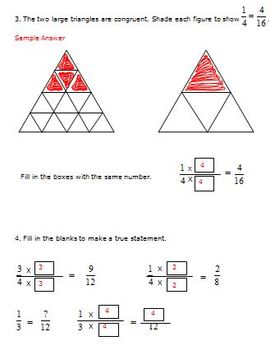# 4th Grade Math - Multiplication/Division, Fractions, 2-D Shapes, Symmetry4th
Subjects
Resource Type
Formats Included
• PDF
Pages
59 pages

### Description

This product focuses on the three critical areas of 4th grade mathematics identified in the Common Core State Standards.

In Grade 4, instructional time should focus on three critical areas:

(1) developing understanding and fluency with multi-digit multiplication and developing understanding of dividing to find quotients involving multi-digit dividends;

(2) developing an understanding of fraction equivalence, addition and subtraction of fractions with like denominators, and multiplication of fractions by whole numbers;

(3) understanding that geometric figures can be analyzed and classified based on their properties, such as having parallel sides, perpendicular sides, particular angle measures, and symmetry.

These activities focus on the following Common Core State Standards.

4.OA.3
4.NBT.1, 5, 6
4.NF.1 , 3, 4
4.G.1, 2, 3

There are 12 activities in this resource. The activities may be used in a variety of ways.

1. End of the Year Review of the Three Critical Areas in 4th Grade Math

2. Review of a Single Unit Taught During the Year

3. Activity While Teaching a Particular Standard or Objective

Each of the activities was designed to be a group activity. Students will discuss information given on a group task card. Then each student will complete a handout.

Please see the preview for samples of the activities.

Included In This Resource:

1.) Teacher Notes

2.) 4 Tasks on Multiplication/Division – Multi-Digit Multiplication, Division with Multi-Digit Dividends, Interpret Remainders

3.) 5 Tasks on Fractions – Equivalent Fractions, Decompose Fractions, Add and Subtract Mixed Numbers, Multiply a Whole Number and a Fraction, Word Problems

4.) 3 Tasks on 2-Dimensional Shapes – Lines, Line Segments, Rays, Angles, Parallel Lines, Perpendicular Lines, Classify 2-D Shapes, Lines of Symmetry

5.) Student Handouts for 12 Tasks

Common Core Math 4th Grade Geometry Activities Angles Shapes Symmetry (4.G)

Common Core Math 4th Grade Measurement (MD) Activities Area/Perimeter

Common Core Math 4th Grade Measurement Activities - Angles (4.MD.5,6,7)

Easter Math Common Core Activities Grade 4 (4.OA.3, 4.OA.4, 4.NF.2)

4th Grade Math Multi-Digit Multiplication CCSS 4.NBT.5

4th Grade Math Activities Division Strategies Multi-Digit Dividends CCSS 4.NBT.6

4th Grade Math - Equivalent Fractions - Compare Fractions - 4.NF.1, 4.NF.2

Created by Hilda Ratliff
Total Pages
59 pages
Included
Teaching Duration
N/A
Report this Resource to TpT
Reported resources will be reviewed by our team. Report this resource to let us know if this resource violates TpT’s content guidelines.